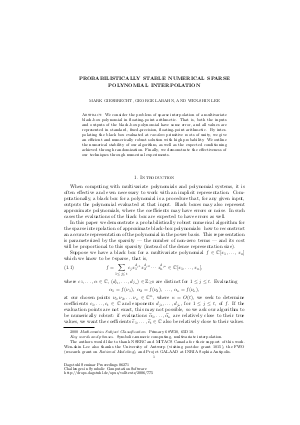Document# Probabilistically Stable Numerical Sparse Polynomial Interpolation

### Authors Mark Giesbrecht, George Labahn, Wen-Shin Lee## File

DagSemProc.06271.14.pdf
• Filesize: 243 kB
• 11 pages

## Cite As

Mark Giesbrecht, George Labahn, and Wen-Shin Lee. Probabilistically Stable Numerical Sparse Polynomial Interpolation. In Challenges in Symbolic Computation Software. Dagstuhl Seminar Proceedings, Volume 6271, pp. 1-11, Schloss Dagstuhl - Leibniz-Zentrum für Informatik (2006)
https://doi.org/10.4230/DagSemProc.06271.14

## Abstract

We consider the problem of sparse interpolation of a multivariate black-box polynomial in floating-point arithmetic. That is, both the inputs and outputs of the black-box polynomial have some error, and all values are represented in standard, fixed-precision, floating-point arithmetic. By interpolating the black box evaluated at random primitive roots of unity, we give an efficient and numerically robust solution with high probability. We outline the numerical stability of our algorithm, as well as the expected conditioning achieved through randomization. Finally, we demonstrate the effectiveness of our techniques through numerical experiments.
##### Keywords
• Symbolic-numeric computing
• multivariate interpolation
• sparse polynomial

## Metrics

• Access Statistics
• Total Accesses (updated on a weekly basis)
0